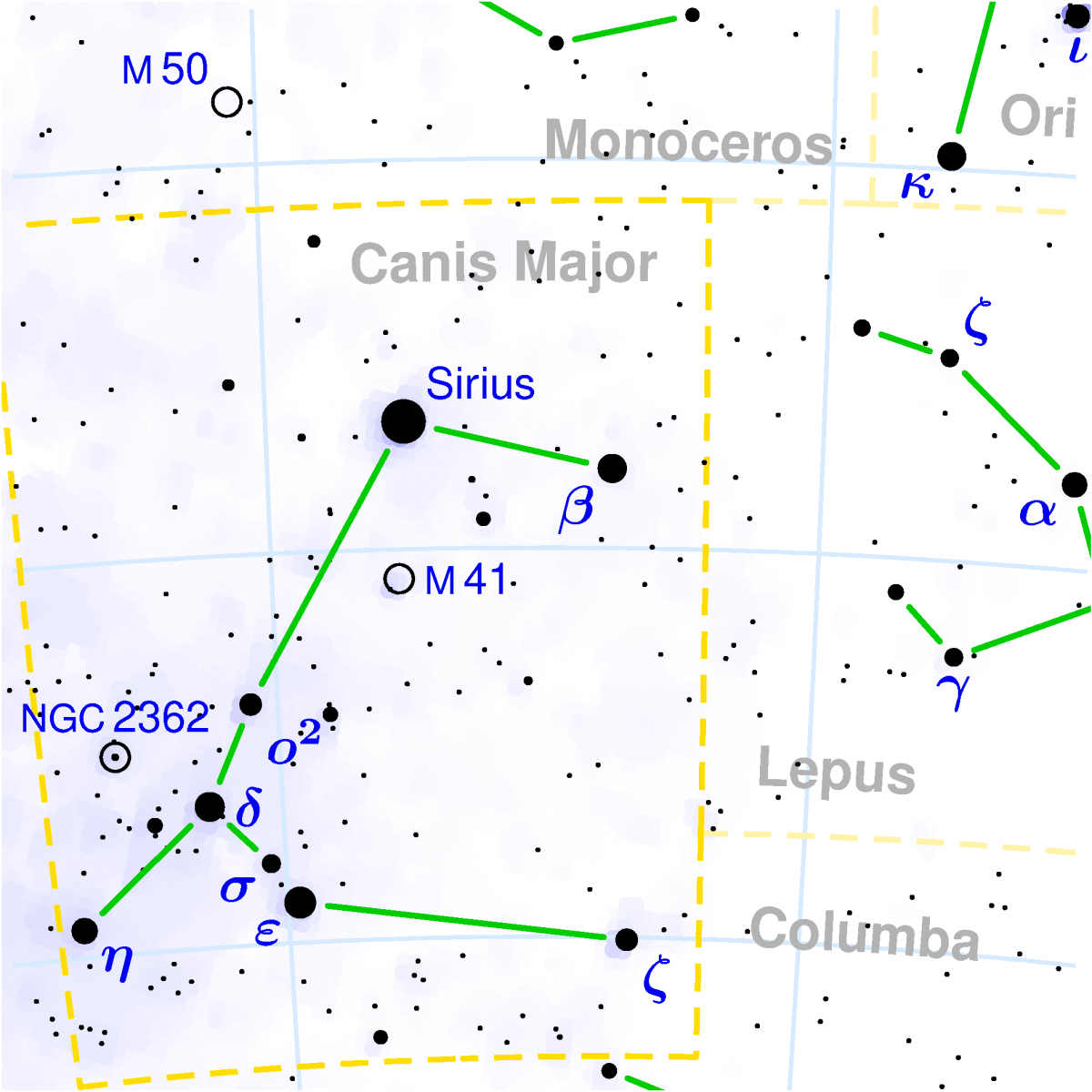# Distant Star

In Astro by Brian Koberlein0 Comments

In the late 1600s Isaac Newton wanted to measure the distance of the brightest star in the night sky, Sirius.  To do this he used a idea known as the inverse square law for light.  Although we now have experimental evidence for the inverse square law, in Newton’s time it was still a hypothesis.

Imagine flash of light from an object. The light goes off in all directions, which means it is an expanding sphere of light.  Since the surface area of a sphere is proportional to the square of its radius, and the light is spread over that surface, the brightness of the light must decrease in proportion.  Thus the brightness of an object is inversely proportional to the square of its distance.  Thus two stars give off the same amount of light, but one is twice as far away, the closer star will appear 4 times brighter than the more distant star.

In observing the night sky, Newton noted that Sirius was about as bright as the planet Saturn.  Observations of Mars by John Flamsteed in 1672 had established that Saturn is about 9 – 10 times farther from the Sun than Earth, so Newton could use that to determine the distance to Sirius.  Sirius and Saturn appeared equally bright, but Sirius was much farther away.

The catch was that Sirius, being a star, emits its own light like the Sun, but Saturn shines by reflecting sunlight.  So Newton had to calculate how much sunlight would reach Saturn.  This is pretty easy to do with the inverse square law, but Saturn also doesn’t reflect all of the light that strikes it, so Newton had to estimate the fraction of light the planet reflects (known as its albedo).

In the end, Newton calculated that Sirius was about 800,000 times the distance of Earth from the Sun, or about 12.6 light years.  The actual distance is about 8.6 light years, so Newton was at least in the ballpark.  His biggest mistake was greatly underestimating the albedo of Saturn, but he also assumed Sirius was the same brightness as the Sun, when it is actually much brighter.

We still use the inverse square law to determine distances, though we use it in combination with other measurements as part of the cosmic distance ladder.  But that’s a story for another time.